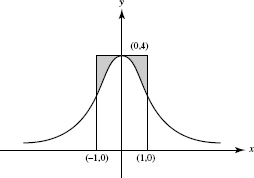# AP Calculus BC Practice Test 22

### Test Information9 questions18 minutes

Calculator Disallowed

1. The graph below shows the velocity of an object moving along a line, for 0 ≤ t ≤ 9.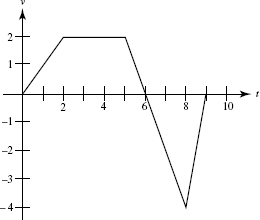The object is farthest from the starting point at t =

2. If x = 2 sin θ, then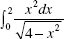is equivalent to:

3.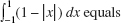4.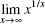5. A differentiable function has the values shown in this table:

 x 2 2.2 2.4 2.6 2.8 3 f (x) 1.39 1.73 2.1 2.48 2.88 3.3

Estimate f ′(2.1).

6. If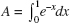is approximated using Riemann sums and the same number of subdivisions, and if L, R, and T denote, respectively left, right, and trapezoid sums, then it follows that

7. If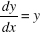tan x and y = 3 when x = 0, then, when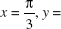8.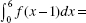9. The equation of the curve shown below is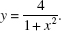What does the area of the shaded region equal?